### 9.1.2 Tableaux for Model Generation with PLNQ

Tableaux can readily be used for model genration.

So for the above reasons we would like to have a technique that derives all literals that a formula implies, that is, we would like to have a model generation procedure . And in fact, we already have one. Look again at the tableaux by means of which we have attempted to prove the entailment ``Mary loves Bill or John loves Mary''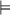``John loves Mary''.

The tableaux is saturated and still open. This tells us that our proof failed, which is correct. But the tableaux contains further useful information: The (green marked) literals on the open branch give us a counter-model for the entailment, namely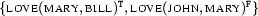. So, as a by-product of the proof, the tableaux has generated a model for its input formula (the negation of the entailment to be proven). We will now use this property of our tableaux calculus directly for model generation. Note that models from now on are signed. The model from above may equivalently be written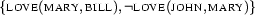.

To find models for a formula, we will simply build a tableaux for this formula with positive polarity (). In the end, each (open) branch of that tableaux will tell us one way of making the formula true - in other words it will contain a model for it.

Let us look at an example, the (admittedly a little unnatural) sentence ``John doesn't love Mary and if John doesn't love Mary then Mary loves Bill'', which we represent by the formula: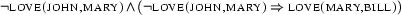which is equivalent to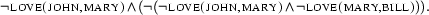Now, which models does this formula have? Before we run our tableaux algorithm, let us give an intuitive formulation of how the models for our formula should look. The first part of our input sentence tells us that John does not love Mary. So, this fact must definitely be reflected in every model. The second part alone (the implication``if...then...'') is true in two kinds of model: Either in a model where John loves Mary or in one where she loves Bill. Now since we already know that John doesn't love Mary, we know that only the second alternative is possible. So there is only one model for our sentence, namely the one where John doesn't love Mary but Mary loves Bill.

Here's the tableaux: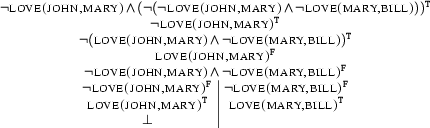This tableaux is saturated and has one open branch, representing a model for the input formula. As you can see the tableaux once had two open branches while it was being constructed. At that time these two branches represented two different models under construction for the input formula. But our calculus found out that the literals on the left branch are contradictory. Hence this branch was discarded as it does not represent a model. So now there remains one open branch in our tableaux, and its literals taken together represent exactly the model we described above: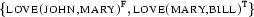Our tableaux calculus made use of the information given in the first part of the sentence while processing the rest. It then automatically made the right decision in discarding the left branch. So it arrived at the same result as we did in our intuitive considerations.

?- Question!

Look at the tableaux again. Which formula expansion resulted in the branching? In terms of models, why does this expansion have to introduce a branching? And why is the closure of the left branch appropriate?

Aljoscha Burchardt, Stephan Walter, Alexander Koller, Michael Kohlhase, Patrick Blackburn and Johan Bos
Version 1.2.5 (20030212)